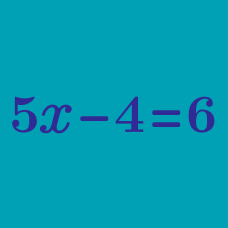Algebra

# Solving Equations

## Intro

If you can solve an equation, then you can determine how far you can travel on a tank of gas, how much money you’ll spend to fill it up, or how many hours you'll drive before you reach your destination. Being able to work through these basic equations will lay a groundwork for solving the menagerie of other equations you’ll encounter on your mathematical journey.

In this chapter, you can expect to see and learn how to solve questions like this one:If you want to jump right in, ​concept quizzes​ train you on a specific technique in equations, while the ​challenge quizzes​ are slightly harder and test your knowledge from a variety of the ideas learned in the concept quizzes.

×